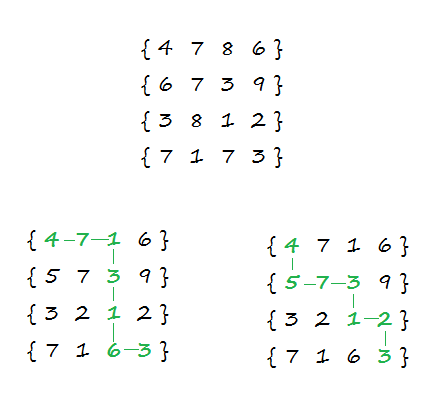# Count number of paths in a matrix with given cost to reach destination cell

Given a M x N matrix where each cell have non-negative cost associated with it, count number of paths to reach last cell (M-1, N-1) of the matrix from its first cell (0, 0) such that path has given cost. We can only move one unit right or one unit down from any cell. i.e. from cell (i, j), we can move to (i, j+1) or (i+1, j).

For example, consider below 4 x 4 matrix where cost is 25. There are two paths having cost of 25.The idea is to use recursion. The problem has an optimal substructure. That means the problem can be broken down into smaller, simple “subproblems”, which can further be divided into yet simpler, smaller subproblems until the solution becomes trivial. The problem can be recursively defined as –

path(m, n, cost) = path(m, n – 1, cost – M[m][n])     (if m == 0)
= path(m – 1, n, cost – M[m][n])     (if n == 0)
= path(m – 1, n, cost – M[m][n])
+ path(m, n – 1, cost – M[m][n])     (otherwise)

path(0, 0, cost) = 1 (if M[m][n] == cost)
= 0 (otherwise)

Here, path(m, n, cost) represents the total number of paths to reach cell (m, n) from cell (0, 0) of matrix M and each path have cost cost.

## C++

Output:

Total paths with cost 25 are 2

## Java

Output:

Total paths with cost 25 are 2

The time complexity of above solution is exponential as we’re doing a lot of redundant work as the same sub-problems are getting computed again and again. As the recursion grows deeper in bigger matrices, more and more of this type of unnecessary repetition occurs.

We know that problems having optimal substructure and overlapping subproblems can be solved by dynamic programming, in which subproblem solutions are memoized rather than computed again and again. Now each time we compute total number of paths with cost c to reach any cell (i, j), we save it. If we are ever asked to compute it again, we simply give the saved answer, and do not recompute it.

## C++

Output:

Total paths with cost 25 are 2

## Java

Output:

Total paths with cost 25 are 2

The time complexity of above solution is O(M x N x cost).

Exercise: Extend the solution to consider diagonal moves as well.(2 votes, average: 5.00 out of 5)Loading...

Please use our online compiler to post code in comments. To contribute, get in touch with us.
Like us? Please spread the word and help us grow. Happy coding 🙂Subscribe
Notify ofGuest
Hitman47

for top-down dp
refer: https://ideone.com/LWeW05Guest

Bottom-up solution using a 3-dimensional array with cost as a 3rd dimension: https://techiedelight.com/compiler/?APw7vujK3cf9TP1WsSzgsva3U6a1
Time Complexity O(m * n * cost)